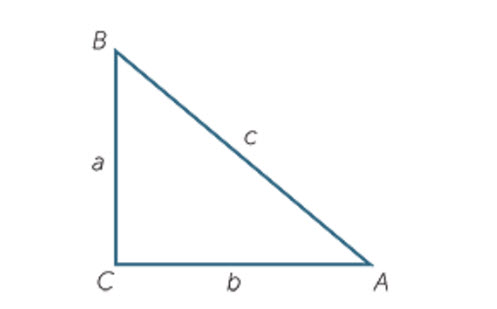Go to website

# Pythagoras’ theorem

This module from the AMSI 'The Improving Mathematics Education in Schools (TIMES) project' is for teachers who wish to consolidate their content knowledge on the topic of Pythagoras' theorem and using it to find the length of sides. There are sections that provide history and context for the topic, as well as exercises and examples of how to present and explain the concepts to students.

Year level(s) Year 8, Year 9
Purpose Content knowledge
Keywords Pythagoras, triangle, right-angle, explicit teaching

## Curriculum alignment

Strand and focus Space
Topics Angles and geometric reasoning, Pythagoras and trigonometry
AC: Mathematics (V9.0) content descriptions
AC9M8M06

Use Pythagoras' theorem to solve problems involving the side lengths of right-angled triangles

AC9M9M03

Solve spatial problems, applying angle properties, scale, similarity, Pythagoras' theorem and trigonometry in right-angled triangles

Numeracy progression Understanding geometric properties (P7)
Understanding units of measurement (P10)
Multiplicative strategies (P9)
Proportional thinking (P7)